Contents

# Contents

## Idea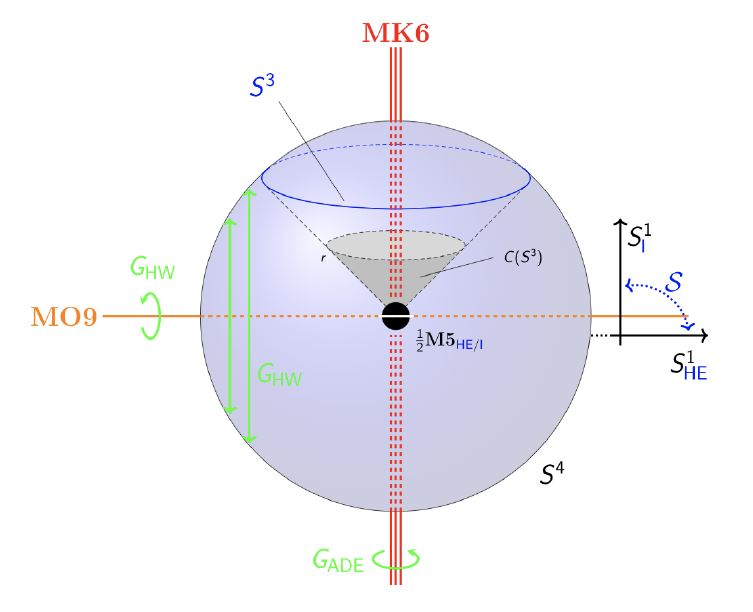$S^1 \!\sslash\! G_{HW} \times \mathbb{H}\!\sslash\! G_{ADE}$

which are, locally, the Cartesian product of

1. the circle orientifolded by $G_{HW} \simeq \mathbb{Z}_2$ as in Horava-Witten theory;

2. the quaternions orbifolded by a finite subgroup of SU(2) $G_{ADE}$.

graphics grabbed from HSS18, Example 2.2.7

For $G_{ADE} = \mathbb{Z}_2$ this subsumes M-theory on K3 times $S^1 \sslash G_{HW}$ (Seiberg-Witten 96)

## Dual string theory perspectives

Under duality in string theory (specifically: duality between M-theory and type IIA string theory and duality between M-theory and heterotic string theory) M-theory on $\mathbb{S}^{1}\sslash \mathbb{Z}_2^{HW} \times \mathbb{T}^{4}\sslash G^{ADE}$ appears through the following string theory-perspectives

The following graphics shows how the three perspectives arise from KK-compactification on three different choices of circle-fibers. Indicated also are the M5-branes and their string theoretic images at NS5-branes/D4-branes with geometrically engineer D=6 N=(1,0) SCFTs (see further below).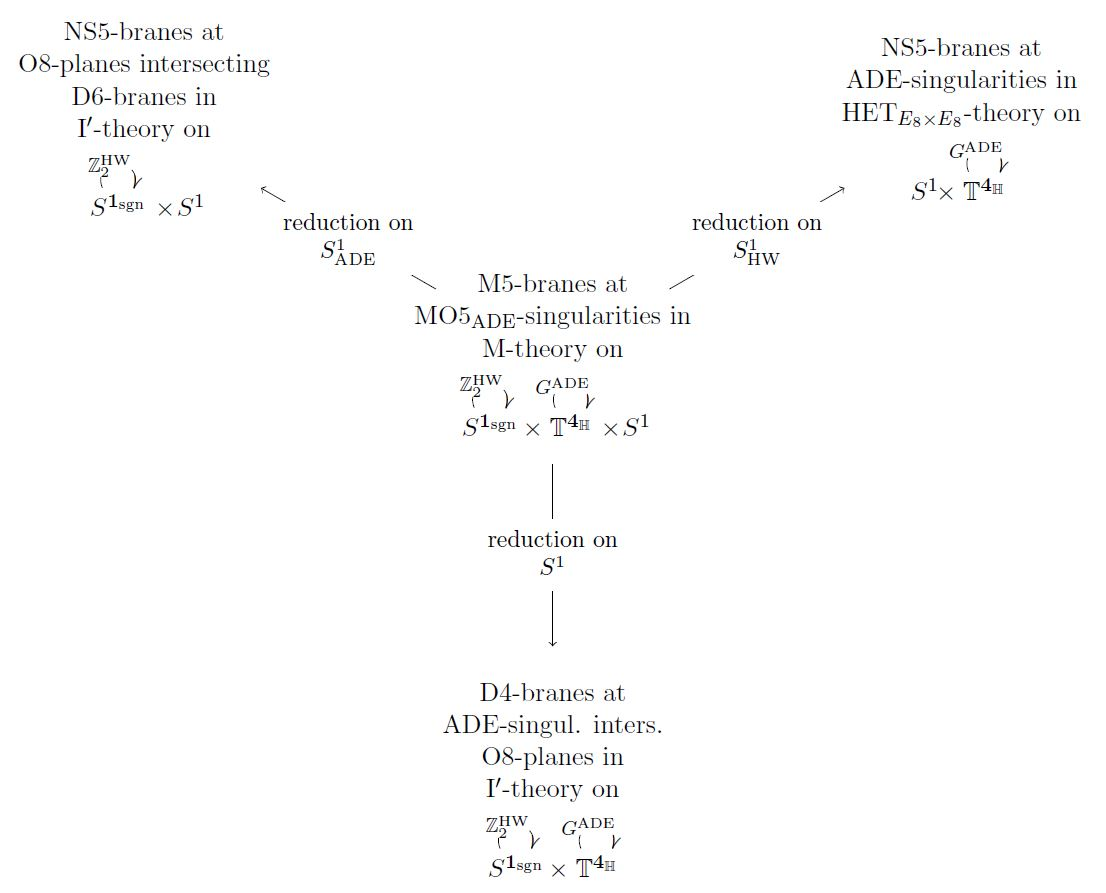graphics grabbed from SS19

### $HET_{E}$-Theory on ADE-singularities

(…)

Horava-Witten theory, hence heterotic string theory, on ADE-singularities $\mathbb{H} \sslash G_{ADE}$

(Witten 99,…)

(…)

### $I'$-Theory with D6-branes on O8-planes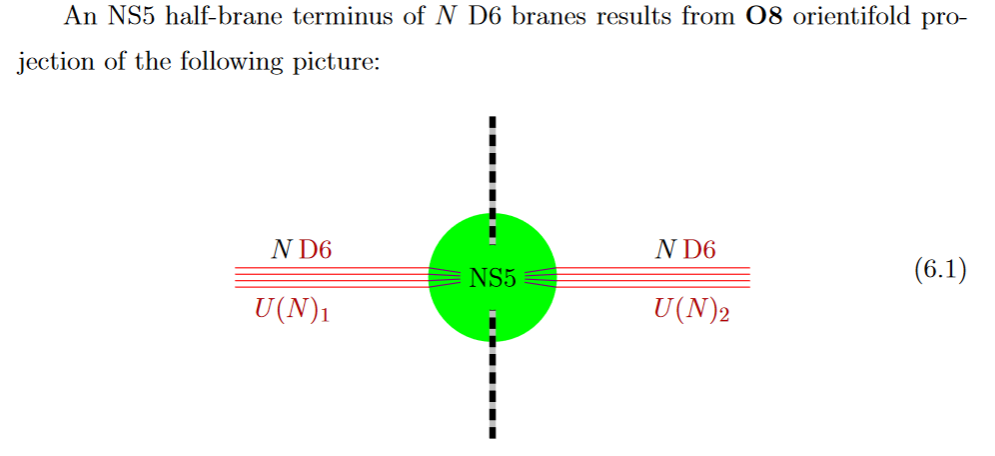from GKSTY 02

If in addition the black NS5-brane sits at an O8-plane, hence at the orientifold fixed point-locus, then in the ordinary $\mathbb{Z}/2$-quotient it appears as a “half-brane” – the half M5-brane – with only one copy of D6-branes ending on it: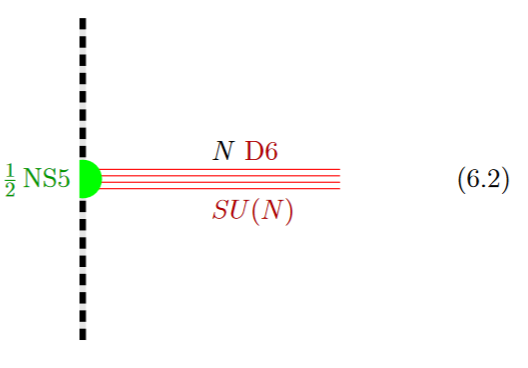graphics grabbed from GKSTY 02

(In Hanany-Zaffaroni 99 this is interpreted in terms of the 't Hooft-Polyakov monopole.)

The lift to M-theory of this situation is an M5-brane intersecting an M9-brane (see at M-theory on S1/G_HW times H/G_ADE):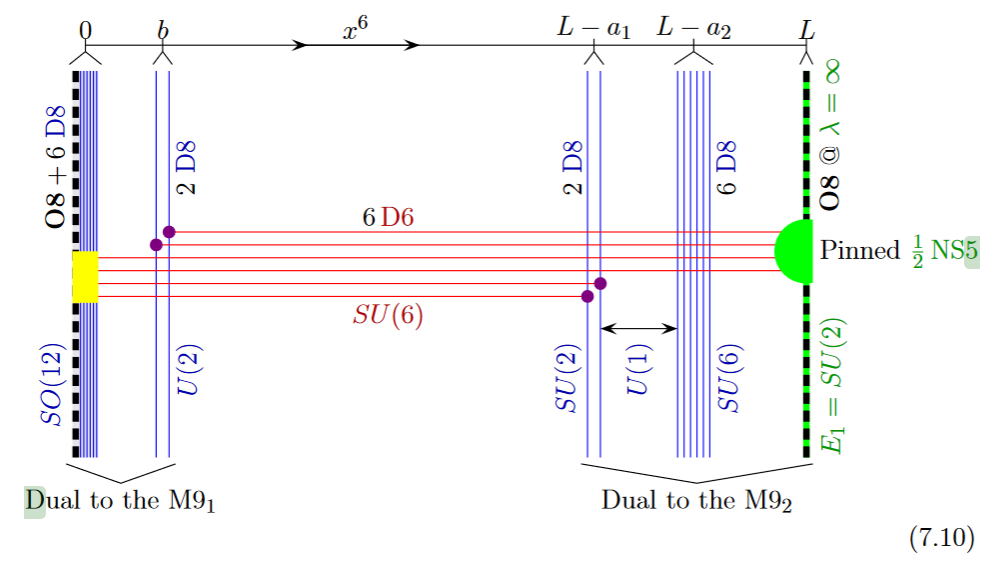from GKSTY 02

Alternatively the O8-plane may intersect the black D6-branes away from the black NS5-brane: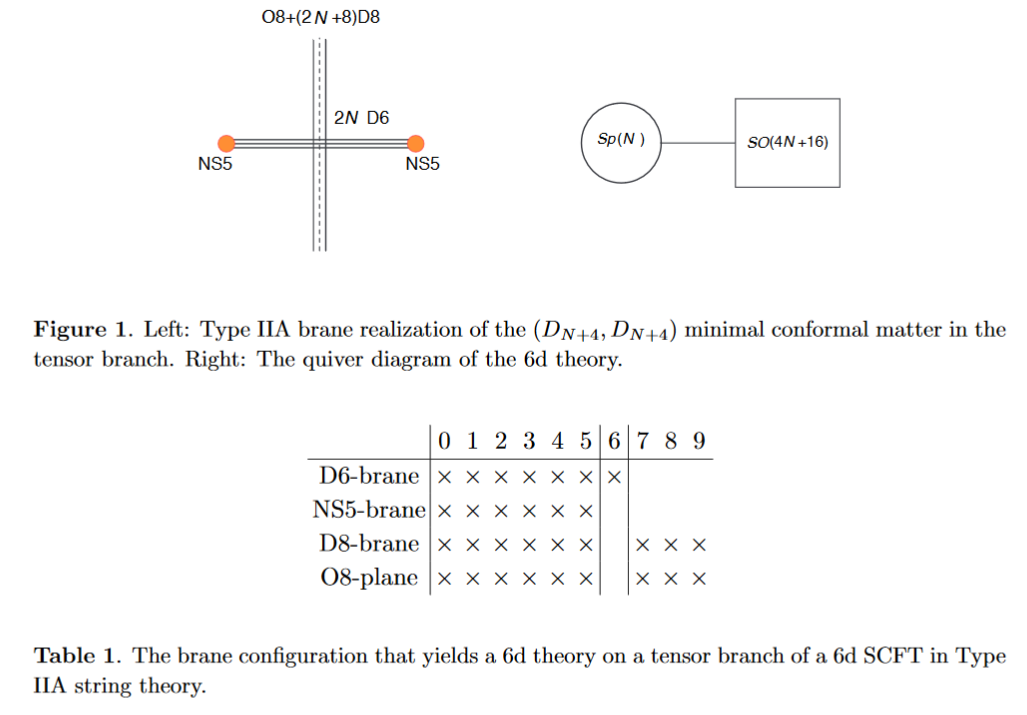from HKLY 15

In general, some of the NS5 sit away from the O8-plane, while some sit on top of it: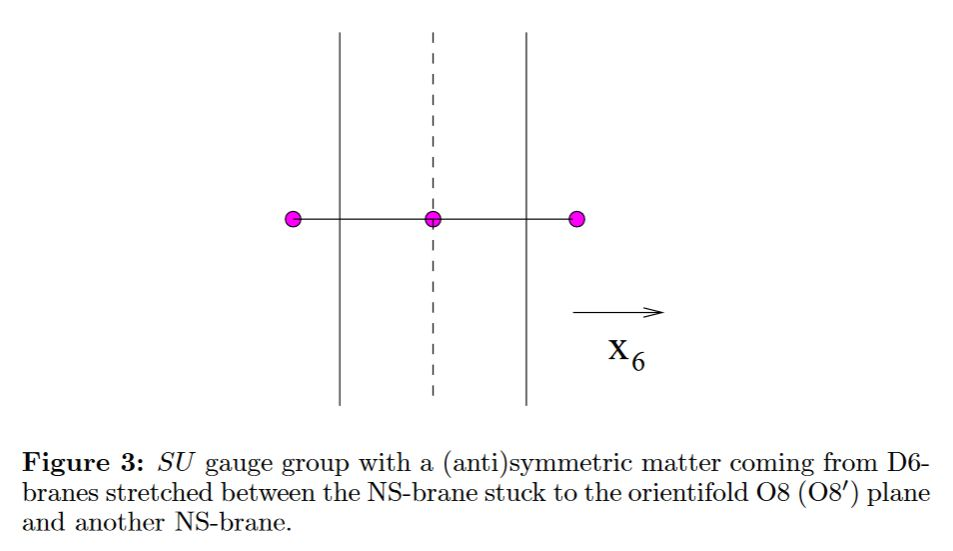(…)

## Geometric engineering of $D = 6$$N=(1,0)$ SCFTs on M5-branes

The M5-branes-configurations as above are supposed to geometrically engineer D=6 N=(1,0) SCFTs.

See the references below, for example DHTV 14, Section 6, Gaiotto-Tomasiello 14, HKLY 15.

### General

The first discussion of this compactification is possibly

in the context of the M-theory lift of gauge enhancement on D6-branes.

The original articles focusing on this situation:

Discussion of heterotic M-theory on smooth K3 originates around

### As $I'$-theory with ADE-singularities

• Oren Bergman, Diego Rodriguez-Gomez, Section 3 of: 5d quivers and their $AdS_6$ duals, JHEP07 (2012) 171 (arxiv:1206.3503)

• Chiung Hwang, Joonho Kim, Seok Kim, Jaemo Park, Section 3.4.2 of: General instanton counting and 5d SCFT, JHEP07 (2015) 063 (arxiv:1406.6793)

### F-theory perspective

The F-theory perspective:

### Geometric engineering of $D=6, \mathcal{N}=(1,0)$ SCFT

On D=6 N=(1,0) SCFTs via geometric engineering on M5-branes/NS5-branes at D-, E-type ADE-singularities, notably from M-theory on S1/G_HW times H/G_ADE, hence from orbifolds of type I' string theory (see at half NS5-brane):

### $SU(2)$-flavor symmetry on heterotic M5-branes

Emergence of SU(2) flavor-symmetry on single M5-branes in heterotic M-theory on ADE-orbifolds (in the D=6 N=(1,0) SCFT on small instantons in heterotic string theory):

Argument for this by translation under duality between M-theory and type IIA string theory to half NS5-brane/D6/D8-brane bound state systems in type I' string theory:

Reviewed in:

• Santiago Cabrera, Amihay Hanany, Marcus Sperling, Section 2.3 of: Magnetic Quivers, Higgs Branches, and 6d $\mathcal{N}=(1,0)$ Theories, JHEP06(2019)071, JHEP07(2019)137 (arXiv:1904.12293)

The emergence of flavor in these half NS5-brane/D6/D8-brane bound state systems, due to the semi-infinite extension of the D6-branes making them act as flavor branes:

Reviewed in:

• Fabio Apruzzi, Marco Fazzi, Section 2.1 of: $AdS_7/CFT_6$ with orientifolds, J. High Energ. Phys. (2018) 2018: 124 (arXiv:1712.03235)

• Amihay Hanany, Noppadol Mekareeya, The Small $E_8$ Instanton and the Kraft Procesi Transition, JHEP07 (2018) 098 (arXiv:1801.01129)